# Alternative Solutions: Practice Test #3

DITW = Do it their way

Trial and Error = pick an answer, play with it, see if it fits what they said in the problem…

Back Door = make up numbers for the variables in the problem, work out an answer that is based on the numbers you made up, then put those made up numbers back into the answer choices, ruling out any that don’t produce a matching answer.

More detailed explanations available in the book…

PRACTICE TEST #3, SECTION 3 – NO CALCULATOR

1. DITW

2. OK to use algebra, but be lazy about it.  Once again, there is no reason to solve for r.  Look at what they ask for and think about how to get there from where you are starting:  take the original equation, double both sides and then add 3…

3. This is NOT a back door problem.  I mean, it could be, but even after you make up a number for a, you still have to know what it means to raise the number to an exponent of 2/3.  It’s the cube root of the square (or the square of the cube root – either way is fine.)

4. This is supposed to be easy algebra, but in fact it is also easy to mess up!  So before you do algebra, read and think:  30 is twice as big as a number, x.  So x is 15.  And now that you know the value of x, checking to see which equation has it right is pretty straight-forward.

5.  Their way: cross multiply, distribute, solve for x, divide by 5.  But you can also do this by trial and error.  For example, if the answer is A then 10 is  x/5.  So x would have to be 50.  Put 50 into the original equation and you see it does not work.  So A is wrong.  Eventually, you will try C: x/5=2 and that makes x=10.  And if you put x=10 into the original equation, it does work!

6. You COULD solve the system, but this problem is actually a throw-back to the kind of thing that used to be on the SAT all the time.  Try adding the equations exactly as they appear.  Look at what you get and compare it to what they asked for…divide both sides by 5 and you will be done.  (To be fair, they do in fact mention this approach in the official solution.)

7. DITW – This is a very basic application of the remainder theorem, disguised in chart form.  If f(4) = 0, then x-4 is a factor.  See page 227 for more detail.

8. Here are two alternatives to their way…

Quickest: apply the slope formula to the points (c,d) and (0,4).

Back door:  make up a k-value to get a line.  I used y=3x+4.  Draw a neat graph to find a point on that line.  Starting from (0,4) I went over 1 and up 3 to get to (1,7).  Use those coordinates as your c and d values, plugging into each answer choice to see which one matches your line’s slope.

9. If the coefficients have the same ratio, then the lines are parallel (or they are the same line).  So set up the proportion  k/4=3/5, cross multiply and divide.

10.  DITW

11. You might discover the relationships more quickly if you make up numbers that fit.  And if it goes wrong on your first try, just try again – you will be solving the problem.  As you fiddle around with the numbers, eventually you discover that x, z, w and t all have to be the same.  And y and u have to be the same as each other but they don’t have to be the same as the others.

12. DITW – they actually give the quickest solution!  I was sure they would take you down a longer path…

13.  You can DITW.  But you can also just let x=1and see what you get.  I think the equation you land on is not that bad, but if you don’t want to solve it, you can try the answer choices and see which one works.  Be lazy…try 3…nope… -3…yep!

14. DITW or complete the square.

15. While you COULD use made up numbers, with no calculator you are probably better off DITW.

16.  This is a clever little problem.  They could have made it a lot harder than they did…before wading in to the factoring needed, why not play with some numbers?  Try x = 1.  It probably won’t work because there is no way they would make it that easy – oh, wait.  It worked.  Never mind.  Oh and look at that: 2 works as well.  How about that.

17.  DITW – and yes, start by clearing the fractions.

18.  DITW

19.  This is a classic two-variable word problem.  So you can DITW if you are comfortable.  But as we discussed (pages 156-158) there are always alternatives.

If you are patient, you can use trial and error even here in a grid-in section.  For example, say the burgers have 600 calories each.  Then the fries have 550 each.  In that case, 2 burgers and 3 fries have 600 +  600 + 550 + 550 + 550 = 2850 calories.  That’s too high.  Lower your guess and repeat.  I know this takes a while, but if you don’t know the algebra, this is one way around it.

But as I have mentioned, you can often think your way out of these.  The burgers have 50 more calories than the fries.  So if we replace the 2 burgers with  2 orders of fries, that means the 5 orders of fries will have 1600 calories.  (That’s the original 1700 minus the 100 we saved by replacing burgers with fries.)  Now we know that each order of fries must have 1600/5=320 calories.  And the burgers have 50 more = 370.

20.  I’m still hungry from solving the last problem.  So for this one, DITW.

PRACTICE TEST #3, SECTION 4 – WITH CALCULATOR

1. DITW

2. DITW

3. DITW

4. Pick an input n-value…say 3.  When you plug it into the function, you are supposed to get 4 as your answer.  Check the answer choices to find the one that works.  If more than one of them work, check another input-output pair.

5. DITW

6. No doubt, you could add these and combine the terms.  That’s probably best.  But you could also use the back door…make up an x value, work out the two expressions, add them.  Then put your x-value into the answer choices.  I’m just saying that would work too.

7. Trial and error…

8.  Once again, we see y=mx + b and we are asked to show that we know that the m-value is the rate of change…

9. DITW

10. DITW – and don’t worry if you have not had physics yet.  All of the information you need is right there in the problem.

11. DITW

12. DITW

13. Again, don’t be intimidated by the physics.  This is a classic back door problem.  Make up t, v and k, then calculate h.  Then stick h, k and t back into your answer choices to see which one gives you the matching v-value.

14. And another classic back door…say h = 3 hours….that’s 180 minutes…at \$.20 per minute, you are looking at a cost of \$36.  Now put h=3 into each answer…

15.  DITW

16. DITW, but they say it in a more complicated way than we need:  you are just looking for the point on the x-axis where the f- function and the g-function add up to zero. So one of them will be above the axis and the other will below the axis by the same distance. That happens at x= -2.

17.  You can look at the supply equation and ask yourself what happens if p increases by 10.  Or you can actually try it: make up a P value.  I’ll try P=20.  I get S(P)=(1/2)×20+40=50.  Now increase the P value by 10 to P=30.  S(P)=(1/2)×30+40=55.  So now we know what happens when P increases by 10.

18. Trial and error –take an answer, put it into both the supply equation and the demand equation.  If the two equations give the same result, you’ve found the answer.

19.  DITW

20. DITW

21. This is just asking if you know what it means to compound interest and that this leads to exponential growth.  Review pages 181-188.

22. OK, I admit that I used algebra on this one — but less messy algebra than their way.  I let 2y be the sum of the other two numbers.  That may seem like an odd choice, but 50% more than 2y is 3y.  So now the sum, 5y=855.  Divide by 5 to get y=171.  And 3y=513.  Also, you could do this by trial and error, but it isn’t really easier that way.

23. Remember, after SOHCAHTOA, it seems that the most important trig fact is the rule about sines and cosines of complementary angles.  Saying that sin(a)=cos(b) is another way of telling you that a and b add up to 90 degrees.  If you realize that, you can find k either by algebra or by trying their answers one at a time. But wait!  Even if you don’t know any of this…you can try each of k-values in the answer choices to find the a-value and the b-value and then just use your calculator to check if sin(a)=cos(b)!

24. They intend for you to set up and solve equations.  But trial and error works too.  For example, if there are 16 students:  3×16=48…and 48 + the 5 leftover = 53 milliliters.  Then, 53 + 21 = 74.  But 74 divided by 4 is not 16.  So move on to another answer choice until you find the one that works.

25.  DITW

26.  You can use trial and error to make sure you get the same slope going from the origin to each of the two given points.  But really, as long as you are doing trial and error, why not just draw a neat diagram?  The one where all three points line up on the same line will be obvious.

27.  Make up dimensions for the rectangle.  10 x 10 is a lazy choice…  Then, increase the length by 10% to get 11.  Try decreasing the width by the percent given in each answer choice.  If the area of the resulting rectangle is 12% less than the rectangle you started with, you have found your winner.  Note: the advantage of choosing initial values that give an area of 100 is that it makes it easier to recognize when the area has dropped 12% — the new area will be 88.

28.  While it would be great if you just looked at this and recognized the correct expression for exponential decay, you can also use the back door.  Say we let t = 20.  The population will drop 10 percent from that original 50,000 to 45,000.  And when you put t=20 into each answer, only one comes out to 45,000.

29.  This is a tough one…but look where you are in the section.  If you knew the numbers in the chart, you could take the number of right-handed females and divide it by the total number of right-handed students.  But they make you work to get the numbers in the chart.  To DITW, you set up a system of 2 equations and two unknowns.  And once again, there are alternatives to that approach:

You could do trial and error, playing with numbers that add up to 18.  Multiply the females by 5 and the males by 9.  If the numbers you get add up to 122, you are all set.

Then there is that think-about it approach.  Suppose the group was all female.  There would be 18 female lefties and 18×5=90 righties.  But we are supposed to have 122 righties.  We are missing 32.  So we need to replace some of the female lefties with male lefties.  Since there are 9 times as many male righties as lefties (instead of 5 times as many) every time we replace a female with a male, we gain 4 more righties.  So to make up that deficit of 32, we replace 8 female lefties with male lefties, leaving 10 female.  10×5 = 50, 8×9=72 and 50 + 72 = 122.

I know this method is tricky.  You would have to practice it to do it with confidence.  But it DOES work!  And if you work through all of the released practice tests, you will certainly find plenty of chances to practice.

30. DITW

31.  Just play with numbers: \$3 + 2 times however many student tickets must be at least \$11 and not more than \$14.  Try numbers until you find one that works.

32. DITW

33. DITW

34. In the “Advanced Basics” section (page 207) I told you that the harder material would have easier questions.  This one is asking you if you know that a full circle is 2pi radians.  You find the fraction we are talking about by dividing:  (5pi/4)/(2pi) and you get 5/8 or .625.

35. DITW

36. DITW – or graph the boundary lines on your calculator and then trace along to find the y-value where the lines meet.

37. DITW  – But once again, stay calm and read.  You are not supposed to already know this law.

38. DITW

OK, this time we were able to find alternative solutions to about 29 out of the 58 problems.

# Alternative Solutions: Practice Test #2

DITW = Do it their way

Trial and Error = pick an answer, play with it, see if it fits what they said in the problem…

Back Door = make up numbers for the variables in the problem, work out an answer that is based on the numbers you made up, then put those made up numbers back into the answer choices, ruling out any that don’t produce a matching answer.

More detailed explanations available in the book…

PRACTICE TEST #2, SECTION 3 – NO CALCULATOR

1. Yes, this is easy algebra.  But even here, there is a minor shortcut: as you do your algebraic manipulations, keep in mind what they ask you to find.  There is no reason to solve all the way for x here.  After you subtract 6 from both sides, double both sides and then add 3.  Or, starting from the beginning, you could double both sides of the equation and then subtract 9!

2. Trial and error.  In fact, the first equation alone quickly lets you narrow down the options to B and C.  B is the one that also works in the second equation.

3. DITW

4. A back door gift!  In fact, try easy numbers:  Say a=1, b=0…that alone does the trick.

5. Trial and error

6. Just know that slope = rise over run and then you can count boxes. The line on the left rises 2 and runs 5.  Since the line on the right rises 4 it must run 10.  Or do trial and error.

7. DITW

8. Trial and error – but start with D because you are looking for the largest possible answer.

D does not work – it makes the angle 45 degrees.  But C works.

9. DITW – although you CAN avoid all of that with a really neat diagram.  Try making your own graph.  Draw it as carefully as you can.  You will see that the intersection point has to be in the neighborhood of (-1, 4).

10. A funny thing happens when you try x = 0…

11. DITW  — and review pages 221-222.

12.  You need pretty nimble algebra skills to do this one their way.  But lucky for you, this is a classic back door problem.  I used F=4, N=6…got R=.4…then went to the answers…

13. DITW

14. Review percents and exponential decay (page 181) – then this is as straightforward as it can be.

15. Another classic back door:  I used x=2, got 8/5 or 1.6…went to the answers…only D matched.

16.  You just need to play around until you find one allowable combination.  If you notice that \$250 + \$750 = \$1000, you can quickly see that 3 of each type is one possibility.  And one possibility is all you need.

17.  The school way might be quickest here:  distribute everything and group the terms, noting that you only care about the “linear” or “x” terms, not the constant term and not the x2 term. But still, this is DITW.

18.  This shape again!  You really need to immediately recognize that this is a pair of similar triangles.  And the little one on top has lengths that are half of the corresponding lengths on the big triangle below.

19.  DITW – but read up on radians (page 215).  This is basically asking if you can recognize a 30-60-90 triangle and then convert the 30 degrees to radians.

20.  When the lines have the same slope, there will be either no solutions or an infinite number of them (if they are actually the same line).  12 is 1/5 of 60 so a=2/5 and b=8/5 but their ratio is 2/8.

PRACTICE TEST #2, SECTION 4 – WITH CALCULATOR

1. You probably can do this by looking and thinking, but if you don’t see it, this is also a back door problem.

2. While this is a classic ratio problem, it can also be thought of as a probability scale-up problem.  The probability of a bulb being inspected is 7/400.  Then multiply that by the bigger sample size (20,000).

3. Trial and error works here if you don’t see the algebra.

4. I was going to say DITW – but their solution makes this harder than it needs to be.  They told you that this is a direct proportion.  So set up a proportion: 8/\$120=20/x, cross multiply and divide.

5. DITW

6. DITW

7. The school way is to factor.  But if you have a graphing calculator, you can graph it and see that the x-intercepts are at 2 and 4.

8. Trial and error works.  But so does thinking!  You’ve lost 2 points on 100 occasions and yet you still have 200 points left.  Hmm…

9. DITW

10.  No tricks — just a function question.  Review pages 121-134 as needed.  They are asking you to find the output  g(3) and then use that as the input to the  function.

11. DITW – but stay calm and don’t get distracted by irrelevant info.  All that matters is the number of words, how many he can read per minute and the hours he will read each day.

12.  Once again, this is a linear rate of change – so it’s b + mx…only this time they are using the letter y to represent the passing time instead of the final amount.  This is a common way that the SAT likes to mess with you – using a different letter than the one you are used to.

13. DITW

14.  They just want to see if you can read the graph!  Start on the y-axis, go over to the graph and then down to the x-axis.

15. DITW

16. This is just like our problem about bus-riders and kids who stay late (page 199).  It is 7/25 and not 7/200 – we are only talking about the students who DID pass the exam.

17.  Do not get distracted by chemistry.  Seriously, all they are asking is can you find a number that is 20% less than 40.  The quick way: 40×.8=32.

18. DITW

19. Median is middle number.  And there are 600 students total. The median is the average of the 300th and 301st kid – but we won’t have to do that.  Here’s why:  starting with the top row of the data, 120 + 140 = 260.  Next row: 80 + 110 = 190.  And the running total is now 260 + 190 = 450.  So somewhere in that second row, we went past that 300th and 301st kid!

20.  This is another probability scale-up.  Both schools have the same probability of 4 siblings: 10/300 or 1/30.  But now you have to multiply by the size of the full population at each school.  When you do that, you get 30 more kids at Washington.

21. A back door question.  Try x = 150 and y = 155.  It’s magic.

22. Yes, the fastest way to do this is to do the algebra.  But once again, if that gives you trouble, the back door is open.  Make up a P and and r, use your calculator to find I. Then put all of those numbers into each answer choice and rule out anything that doesn’t come out right.  More magic.

23. I make my physics students do this kind of thing all the time.  Look at the equation and ask yourself:  what happens when we change the value of r?  Since r is in the denominator, making r bigger makes the result smaller.  And since r gets squared, whatever you do to r also gets squared.  Now look at what they told you: observer A measured 16 times as much intensity.  They used 16 there for a reason: 16 is 42.  It must be that B is 4 times further away than A. So A is ¼ as far away as B.  I know that this takes thinking and concentration, but it is easier than their way.

Wait!  Still not convinced?  You can also do this one by trial and error.  But you have to be brave about making up numbers.  Make up a P value.  Then make up a distance for B.  Then, to try answer choice A, make up the distance for A ¼ of what you made up for B.  Plug them both into the formula and see if Observer A’s intensity comes out 16 times as big as B’s.  If it does, you are done.  If not, try another answer.  But it does.

24.  DITW – review page 168 for help with completing the squares.

25.  Another back door!  Just make up numbers so that a + b = 0 and then plot the points neatly on a graph.  You will see a positive slope every time.

26.  DITW – but really, all they are asking is do you know how to read a graph!  Review pages 127-128 if you are shaky.

27.  Just checking if you know that rate of change is also slope!

28.  This is MUCH less work than you might think!  The line they are asking for is downhill and steeper than 45 degrees.  So of -3 and -1/3, the slope has to be -3.  That narrows it down to A and B.  But we also know that the line has a positive y-intercept.  So much for A.  It’s B.

29.  While this one does have a clever, “math-y” solution, there are two less-clever solutions available.  You can set ax2+b=3 and then use trial and error.  You put in their a and b values and see if you get a positive value for x2

Or, you can use your graphing calculator!  Graph y=3and y=ax2+b using their a and b values one at a time.  If the graphs intersect at two points, you are done.

30. DITW

31.  I know there is an easier way, but for some students, trial and error is actually better.  Try 10 years…you get 15 feet.  Too small.  Try 20 years.  You get 30 feet.  Too big. 15 years?  22.5 feet…getting close now…14 years?  And we have a winner.

32. Again, if you need it, trial and error will work.

33. This is a perfect example of “The Case of the Missing Constant” (see page 126).

They are telling you that when x=3 is the input, you get f(3)=6 as the output.  So put x=3 into the rule for the function, set the output equal to 6 and solve for b

34.  Use algebra if you like, but this time even the most devoted algebra students have to admit that playing with numbers is faster.  You just need two numbers that add up to 250 and one is 40 more than the other.  How about 150 and 100.  Ooooh that’s almost it.  OK, 145 and 105?  Done.

35.  And now we have yet another example of y = b + mx, dressed up in different letters.  Jane must have started with \$15 and must be depositing \$18 per week.

36.  DITW

37. DITW – but while this is certainly hard (and look where we are in the section!) it may be easier if you think of this as a function problem.  They want you to find next year’s population as the output when you know this year’s population as the input.  Then, to answer their question, you have to take the output from after one year and use it again as the input to find the next year after that one.

38. DITW

Once again, around 36 of the 58 had some alternate path available.

# Alternative Solutions: Practice Test #1

DITW = Do it their way

Trial and Error = pick an answer, play with it, see if it fits what they said in the problem…

Back Door = make up numbers for the variables in the problem, work out an answer that is based on the numbers you made up, then put those made up numbers back into the answer choices, ruling out any that don’t produce a matching answer.

More detailed explanations available in the book…

PRACTICE TEST #1, SECTION 3 – NO CALCULATOR

1. You can just try the answer choices one at a time…

2. DITW

3. Back door!  Make up your own m and p

4. Recognize that this is y = b + mx…the b is the starting value.

5. This looks like a back door problem and I guess you could do that, but this time, I agree that it is actually much faster to do the algebra: distribute the minus sign and group the terms…so DITW

6. Either recognize that y = b + mx, and m is the rate of change…or to see how much the boy grows each year, just make up a number for a, plug it in and then plug in a second number, one year older.  For example, try a=10 and then a=11.  The difference is how much he grew in a year.

7. DITW

8. If you see the algebra, it’s quick.  But making up numbers is even quicker. I used a=4, and b=2…but any numbers that fit will do.

9.  Use trial and error!  But be lazy: check the easier equation first.  Only one choice works.

10.  This is an example of “the case of the missing constant” (see page 126). But if you do notice that the function is an even function, you can go right to the answer: g(4) = g(-4) = 8.  So “their” way is quicker but requires more insight.

11. Trial and error would work, but faster to DITW

12. Draw a neat diagram with a line through the origin that rises one and runs seven.  It will be obvious that only one of the given answers could possibly lie on that line.

13. This is a gift to students who know the back door!  Say x = 6…work out the fraction, common denominator and all that…then put  x = 6 into each answer.

14.  Faster if you know the laws of exponents.  But if you are shaky…back door!

I tried x=4, y=0.  Took a little while to show that 212 = 84, but it works.

15. This is a tricky one whether you DITW or mine…First notice that if you start to foil out the expression, you find that ab=15.  And they gave you a + b = 8.  You could solve that system algebraically, but I prefer to look at the equations and try to make up numbers.  Seriously, the first numbers I tried were 5 and 3!  (It can be a=5, b=3 or the other way around.)  If you put a=5 and b=3 into the original expression and then go ahead and foil it all the way, you get 41.  You don’t need the other possible value to know that the answer is D.

16. Algebra or just a quick trial and error

17. These are two similar triangles – it’s one of the classic examples.  Fill in the lengths they give you and you will see that the bigger triangle’s sides are twice the length of the smaller…

18. DITW

19.  The sine of an angle is also the cosine of the complement.  So the answer is 4/5!  And by the way, if you have a hard time remembering that rule, it may help to think of “cosine” as short for “complement’s sine”!  Works for tangent and cotangent as well…

20.  DITW

PRACTICE TEST #1, SECTION 4 – WITH CALCULATOR

1. DITW

2. Like a “missing constant” problem, you could plug in their values for x and y, solve for k and then plug in the new x value.  Or you could recognize that if y=kx, then x and y are directly proportional – set up ratios, cross multiply and divide.

3. Know the rules about parallel lines and transversals: pairs of angles that are not congruent are supplementary.  180 – 35 = 145.  (See page 95 for a review)

4. Algebra is quick here, but this can also be solved with trial and error:  Be lazy though – start with the choices that are easily divisible by 8.  D does not work, but check out C:  If 8x = 16, then x = 2…and 16 + 4x comes out to 24 which is 10 more than 14.  How about that.

5. DITW – what else could it be?  There is only one data set with a clear downward trend.

6. DITW

7. This is not really algebra.  Just add up the heights of the bars on the graph.  It adds up to 27.5.  But they tell you that the total is actually 27,500.  So the numbers on the side of the graph must be “thousands.”  The “official” solution says the exact same thing but makes it look more algebraic.

8. You can do this by trial and error:  carefully try 0, 1 and 2.  None of them work.  So it has to be D.  You can also do this by thinking:  the smallest value you can have for the part of the expression with absolute value bars is zero.  Then they add 1 to that value.  So it can never add up to zero.

9. Back door!  Say t = 10  Plug in and get a=1062.8.  Put that into each answer – only A comes out to 10.

10.  Trial and Error – put each answer into the equation they gave you…

11. Trial and Error.

12.  Really helps if you just list out all of the data:  3,3,5,5,5,5,6,7,7,9,9,9 – now finding the average is just a matter of adding them up and then dividing.  And by the way, had they asked for the median, a list like this would make that very easy too.

13. DITW

14. DITW

15. This is another example of y = b + mx.  The only difference is that the vertical axis is labeled with a C instead of the customary y.  But the intercept is still the starting amount…

16.  …and the equation is still y = starting amount + slope  × run.  That the slope is 3 can be determined just by counting rise over run on the graph.  But don’t just count boxes – look at the scale of the graph!  Otherwise, you get ¾ which is wrong.

17.  They are just asking you to find the INPUT (x-value) that gives the lowest OUTPUT (y-value).

18.  DITW

19.  Yes, you could do algebra here.  Trial and Error also works:  for example, if they sold 77 salads, that would be 77 × \$6.50 = \$500.50.  So they would need to take in another \$336 from drinks.  At \$2 each, that means 168 drinks.  But 168 + 77 is more than the 209 items they sold.  Now you try it with choice B.  You are in for a happy surprise.

20. Classic back door.  And like most back door problems involving percents, it’s a good choice to make the starting value \$100…The 20% discount knocks the price down to \$80.  With 8% sales tax, what you pay is p = \$86.40.  Put that value into each answer choice…wait for the magic.

21. DITW

22. DITW

23. DITW – annoying and kind of tedious, but what are you gonna do?  Read the chart, find the ratios, pick the one closest to human resources.

24.  OK, this one makes me laugh.  You are supposed to use the center and endpoint of the radius to find the length of the radius and then write the equation of the circle in standard form.  But there is a lazy work-around:  that endpoint they give you does have to be a point on the circle.  So you can just put that point’s x and y values into the equations given in each answer choice.  Only one choice works.

25.  Trial and error – plug in the choices, pick the one that gives a height closest to zero. (When you are on the ground, your height above the ground is zero.  That’s the only physics knowledge you needed to answer this.)

26.  If you don’t want to do the algebra, go to each answer choice, find 20% and add it on.  When you get to choice B, you will see that you end up with the correct number of pears.

27.  This is like a scale-up probability problem.  The sample regions have an area of 1 square meter.  The full region is 100 square meters.  So you just need the answer that is 100 times the average of the data points.  That average is in the neighborhood of 150…

28. DITW

29. DITW – but review the section on the remainder theorem (page 227).

30.  Well, in theory you are supposed to complete the square.  But look at the graph: the vertex is clearly in the neighborhood of (1,-16) and there is only one answer choice where both of those appear directly as constants.

31. Just pick a number between 12 and 18 – let’s say he does 15 per hour.  Divide 72 by 15 and move on.

32. DITW

33. DITW

34. DITW

35. DITW

36. You are looking for the value that makes the denominator zero.  This is one of those questions where you can make use of your calculator.  If you have a TI89, just set the denominator to zero and use the F2 command to solve that equation.  Or with any graphing calculator at all, graph the equation and then trace along the graph to find where the function equals zero.

If you decide to do this one by algebra, a neat trick is to replace x-5 with a new variable, say m.  If you do this, you get an expression that is easy to factor.  But remember to go back and solve for x.

37.  Review page 74 to see the quick way to calculate percent changes:  the base in that expression is 1.02 – that gives you a 2% increase each year.

38.  Review percents and exponential growth (page 181).  Then you will see that all you need here is to calculate (100)(1.02)10 and 100(1.025)10 and then subtract.

OK, that’s all for the first practice test. If you are keeping score, I believe that for 36 out of the 58 problems, there was an alternative method available.

# Polar Graphs in Interactive Physics

This week, I find myself having to remember how to graph in polar coordinates.  I am talking about cardioids, rose petals, lemniscates and the like.  It’s been a while…

There are many websites that will draw these for you.  Desmos has polar mode.  But I have not yet found a website that will show the graphs being constructed.  But I remember reading a very nice explanation that (I think) was in one of Howard Anton’s calculus texts.  If I am remembering this right, he said something like:

Imagine a clock with one hand that rotates around, connected to the x-y plane at the origin.

Then, imagine an ant (Anton’s Ant!) walking along that clock hand as it rotates.  The ant’s distance from the origin is given by the function r=f(θ).

As the clock arm rotates, the ant moves in an out along the arm.  The location of the ant traces out the graph of the polar function r=f(θ).

And note that if the radius is negative, that just means that the ant has walked “the other way” along the clock arm.

For example, these are three  pictures showing the clock arm and the ant at successive times as we graph the cardioid r = f(θ) = 2 + 4 cos (θ):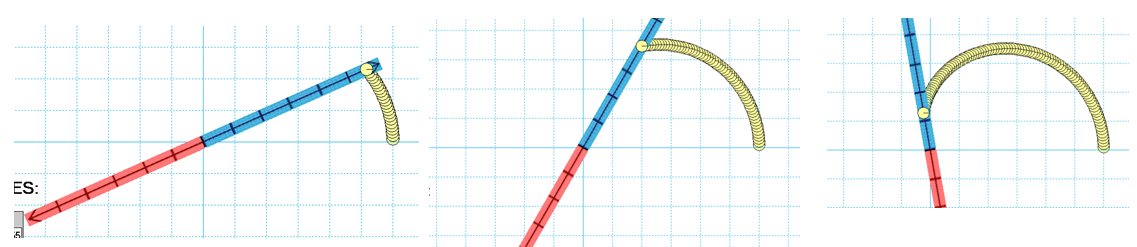I don’t know how to make my own applets, but I do know how to use Interactive Physics as a work-around.  It’s not as good as a real applet.  You can change parameters easily but if you want to change to a new function, that takes more work.  And if you want to share with students and colleagues, they need the software, which is kind of pricey.  (Though there is a way to get student versions quite reasonably.  Email me if interested…totally legal!)

In any case, one thing you can do is export your experiments as videos.  You lose the ability to vary parameters but at least you get the flavor.  So that’s what I did…

A CARDIOID with an inner loopA ROSE PETAL with 5 loopsIf you do happen to have the Interactive Physics software package and would like the source files for these demos, post a comment or send me an email.  That way, you can run these yourself on your own computer, changing the parameters to make discoveries.

Or just leave them to run for as long as you feel like.  They are very soothing to watch.

# 3D visualization, Geometric Probability and 538’s Riddler #2

Hello all…sorry the posts are coming at such long intervals.  I am teaching a new class this year — multivariable calculus! — and it is taking up lots of time as I try to remember/recreate/invent some level of understanding, having last taken the course 30 years ago.  But I am having a lot of fun with it, teaching a small group of fine (and patient) students, learning a lot.

And speaking of things that come at long intervals…here is a puzzle that was posted on 538.com in their new feature: “The Riddler”.  This was from week #2:

You arrive at the beautiful Three Geysers National Park. You read a placard explaining that the three eponymous geysers — creatively named A, B and C — erupt at intervals of precisely two hours, four hours and six hours, respectively. However, you just got there, so you have no idea how the three eruptions are staggered. Assuming they each started erupting at some independently random point in history, what are the probabilities that A, B and C, respectively, will be the first to erupt after your arrival?

The full post is at:  http://fivethirtyeight.com/features/which-geyser-gushes-first/

and a complete solution is in the next week’s post:

http://fivethirtyeight.com/features/how-long-will-your-smartphone-distract-you-from-family-dinner/

But what I want to discuss is a different way of thinking about the solution than the one posted at 538.  I want to use geometric probability.  [If you want to solve this on your own, spoilers ahead.]

Suppose the only geysers were A and B.

If you have just arrived, Geyser A will erupt in x hours, where x is between 0 and 2. And Geyser B will erupt in y hours, where y is between 0 and 4.  Intuitively, it seems likely that y will be greater than x.  One way to see this is to use geometric probability.  Think of every possible outcome as an ordered pair.  Then, all possible outcomes can be represented as an area on the coordinate plane.  I used Desmos.com to graph the region and also the line y=x, or more precisely, the shaded half-plane y>x.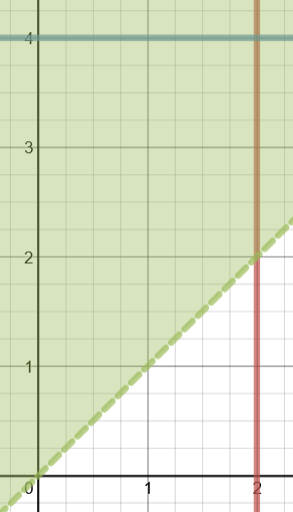Geyser A will erupt first whenever y>x. So the probability we seek is the area of the part of the box above the x=y line divided by the total area of the box.  You can just about count boxes to determine that this occurs 6/8 (or 3/4) of the time.

OK then.  Now let’s bring Geyser C back into the problem…

From the moment you arrive, you know that Geyser C will erupt in z hours, where 0<z<6.  But you can still use geometric probability.  Each outcome can be thought of as an ordered triple (x,y,z).  The space of all outcomes is now a 2 x 4 x 6 rectangular solid.  And if you are looking for the probability that Geyser A erupts first, you want to slice that solid up with the planes x=y and x=z.

I don’t know if Desmos can do this kind of thing.  But fortunately, Paul Seeburger’s Multivariable Calculus Exploration applet can be used to visualize this problem.  You can find the applet here:

http://web.monroecc.edu/manila/webfiles/calcNSF/JavaCode/CalcPlot3D.htm

Note: on my computer, I can only open this page in Firefox.  YMMV.

Here is the solid that represents the set of all possible outcomes: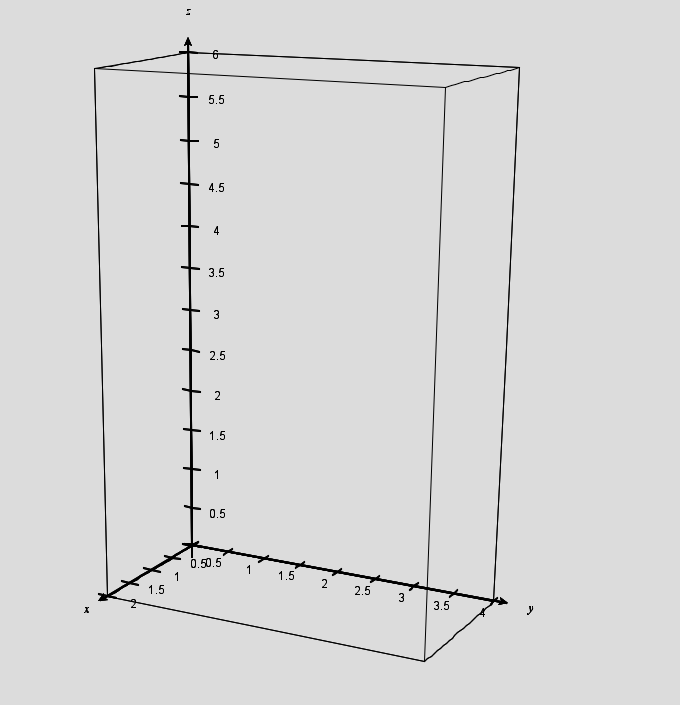And here is that same solid, cut by the planes x=y and x=z: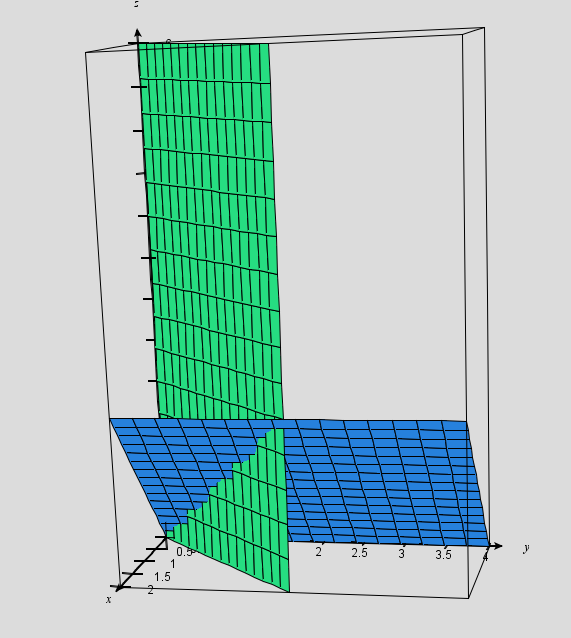We need to find the volume of the part of this box “to the right” of the x=y plane and “above” the x=z plane. It’s easier to see what we are looking for if you rotate the diagram to view it “from behind”:In this diagram, the volume defined by points where y>x and z>x can be broken into four chunks.  I’ve added the x=2 plane and the z=2 plane to help you see them.

The rectangular solid on the upper left: V = 2x2x4=16

The triangular prism in the front on the lower left: V = (1/2)x2x2x2=4

The triangular prism in the front on the upper right: V = (1/2)x2x2x4 = 8

The front half of the pyramid on the bottom right: V = (1/3)(2)(2)(2) = 8/3

(If you are having trouble seeing the pyramid: in this view, we are looking through its 2×2 base, looking toward its vertex which is 2 units away.)

The probability of Geyser A erupting first is the sum of these volumes divided by the total volume of the box, or:

(16 + 4+ 8 + 8/3)/(2x4x6) which reduces to 23/36.

Using a similar method but with different cutting planes, you can find the pictures that will give you the probabilities of Geyser B or Geyser C erupting first.  And you do get the same answer that is posted on the Riddler’s webpage.

You can post questions (or ask me in class) if you need help with those last two…

# “You keep using that word…”

The word is “exponential”.  It’s been showing up on my students’ tests and in their lab reports.  And, like Inigo Montaya, I have to say:  I do not think it means what you think it means.

In particular, it does NOT just mean “curving upward”.  When you state that a graph is “exponential”, you are making a very specific claim about the structure of that graph and the mathematical relationship it depicts.  Some curved graphs turn out to be exponential.  But not all of them.  (And in fact, not this next one.)

What got me started on this year’s version of this rant:

Here is a position vs. time graph from my student’s first test of the year.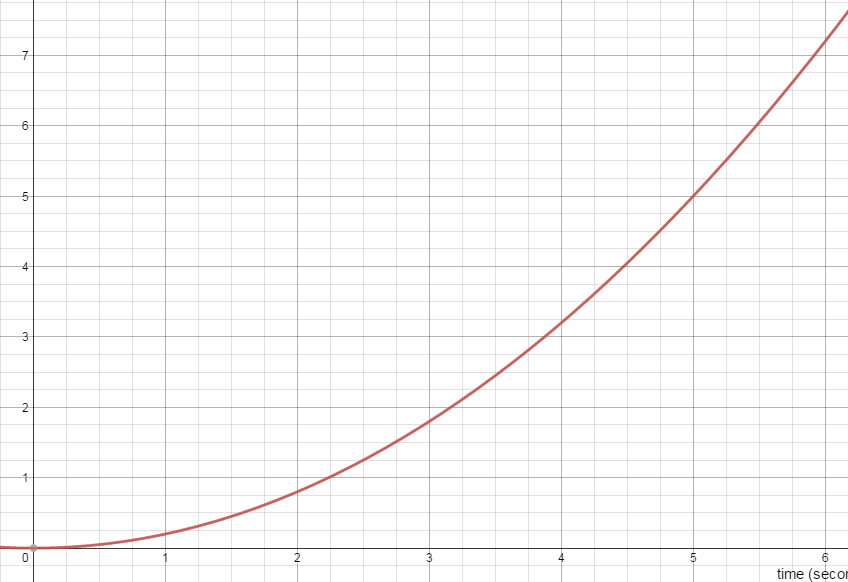“Between t = 0 seconds and t = 6 seconds, was the car accelerating?  How do you know?”

I am hoping for (and giving full credit for) answers like:

Yes.  The slope tangent line is getting steeper.

OR

Yes.  If the velocity were constant, the graph would have been linear.

But I am also seeing a surprising number of papers that say:

It’s accelerating because the graph is increasing exponentially

I don’t take off credit (yet) but I do wish we could sort this one out.  So let’s try.

A Function is Exponential…

only if it can be written in the form: y=a·bx

where a and b are constants and x is the exponent.. And as long as b is greater than 1, the graph of the function will in fact curve upward.  For example, here is the graph of a function that is in fact exponential.  I don’t want to reveal what function it is just yet.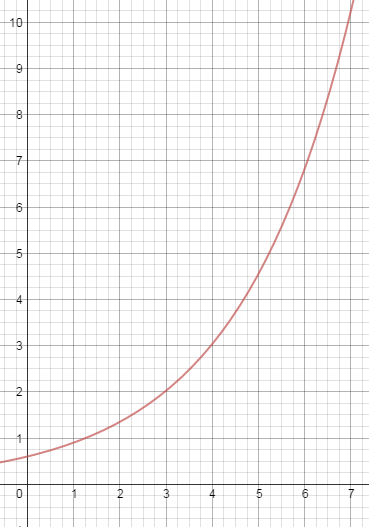And here is a quick way to see if the function is exponential:  look at how much it increases in equal x-intervals.  A linear function will increase (or decrease) by the same amount in each interval. But an exponential function will increase (or decrease) by the same factor in each interval!

So now let’s take a look at the values of this function at regular intervals.  In this case, I’ll just use Δx =1 and I’ll read the values off the graph as best as I can.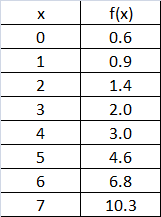As you can see, as x increases, the function increases, but not by equal amounts. That’s ok — we weren’t expecting equal increases.  The function is not linear.

But if you calculate the ratio of any two successive values, you do see that the function is increasing by (nearly) the same factor every time!  (You can trust me, or you can actually do the math.) This means that you are justified in suspecting that this curve really is “exponential”.  And you can figure out the equation by inspecting the graph and chart.  I’ll call that a puzzle — leave your answer in a comment (or if you are my current student, you can also post your answer in our class stream).

So let’s take another look at the first graph:Let’s check on how much this graph increases each interval.  Here’s the data: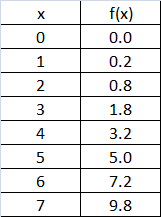Some things to notice:

1. The values increase, but once again, not by the same amount each interval.  This one is not linear either.

2. The ratio of successive terms is NOT constant.  Try it and you will see.  This graph is NOT exponential.

But there is a pattern to be discovered here.  Instead of finding the ratio of successive terms, try calculating the difference.  You will discover a pattern, one that is true for “quadratic” functions.  Tell me about it in the comments if you like.

tl/dr?

Bottom line: curved does not automatically mean exponential.  Exponential means increasing by equal factors in equal time intervals.

# Another look at “Logarithms!”

OK, even I will admit that the logarithm rules do not seem to be infused with joy.  But once you know them, there is a way to apply them that my physics students seem to get a kick out of.  It’s a little exercise I learned by reading Professor David Mermin’s essay, “Logarithms!” in his excellent book, “Boojums All the Way Through” (Cambridge University Press, 1990).

The goal of the exercise is to be able to determine common (base-10) logarithms without a calculator.  At the time Mermin wrote, scientific calculators were actually not so common.  Now, everyone has access to them and Google will calculate them for you as well.  But that’s not the point.  It feels almost magical to be able to do this stuff without a calculator.  I would say it’s a hoot, definitely worth of the exclamation point Professor Mermin put in the title of his original article.

Here’s the basic plan.  Start by observing some coincidences, some “almost” equalities involving powers of small prime numbers and small powers of 10. Then use the logarithm rules to deduce the values of the logarithms.

[All of the examples that follow are from Mermin.]

For example, it happens that…

210=1024 and 103=1000

So we would be almost correct saying 210   = 103.

Then we could take the log of both sides, applying the power rule for logs to get:

10log 2 = log 1000

but we know that log 1000 = 3.

So 10log 2 = 3 and that means log 2 = 3/10 =.3

I have never presented this to a class without at least a handful of kids grabbing their calculators to see if we are close.  Their calculators tell them that log 2 = .3010 and I hear “Whoaaa!” (but I teach an impressively nerdy group).

Now what about log 3?  We need another coincidence, another “almost” equality.

While it is true that 32=9 which is almost 10, we can come closer with

34 = 81 which is almost 80.  This is helpful because 80 is also 10×2.

Starting from 34 = 10 × 23

we can take the log of both sides and actually enjoy ourselves as we apply the log rules to get:

4 log 3 = 1 + 3 log 2

And we already know log 2 — it’s (roughly) .3, which we can substitute in to get

4 log 3 = 1 + 3 × .3 = 1.9

log 3 = 1.9 ÷ 4 = .475

And when check out log 3 on your calculator, you see .4771….whoaaa.

We can get log 4 immediately from the fact that

Log 4 = log 22 = 2 log 2  ≈ 2 × .3 = .6  (whoaaa)

and log 5 = log (10 ÷ 2) = log 10 – log 2 ≈ 1 – .3 = .7 (Can I get another whoaaa?)

OK, I think you see the flavor of how this works now.  We just need to keep finding helpful coincidences…

Like 72 = 49 ≈ 50 = 100 ÷ 2.  Or  112 = 121 ≈ 120 = 10 × 2× 3.

These starting points expand our collection of approximate logs.  But you have to know your log rules to build the collection.

Mermin does not stop here.  He writes:

“Those whom the schools have taught to hate numbers will probably want to stop after this preliminary warmup, but the real fun is only beginning.”

He then goes on to improve on all of these estimated logs by constructing systems of equations built on closer, dazzling coincidences.  I recommend the essay and in fact the whole collection.

# Lost In Translation — At Labyrinth Books in Princeton

In Advanced Math for Young Students, there is one section where I introduce a method to help students when they are stuck at the beginning of a word problem, not yet seeing how to translate the problem into the language of algebra.  Well, I have developed a workshop about this method and have been invited to present it at Labyrinth Books on Nassau Street in Princeton.

The presentation will be on June 11, 2015 at 5:30 pm.  If you are in the area, stop on in. But if not, check back here in mid-June.  I will post more about the method and include a link to the PowerPoint slides.

For more on the presentation:

# For Small Angles Only

Two Surprising Trigonometric Almost-Equalities

Let’s warm up with an old math joke I first heard back in my own freshman year.  I have always thought it was funny even though I am not sure I get it.  But my students all LOVE my math jokes, so here goes: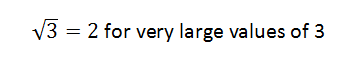I think it is meant to make fun of the way we sometimes do math in physics. For example…

When we are studying Young’s two-slit interference experiment, there comes a point when my students are asked to accept that for small angles, two things that they know are NOT equal can be sort-of, kind-of treated as equal:

sin(θ) ≈ tan(θ)

And when we are studying simple harmonic motion, I ask them to accept another one:

sin(θ) ≈ θ

That one is also only “true” when the small angles are measured in RADIANS (as you will see if you bravely read on).

Once you accept these two things, you can go on to derive other interesting rules about interference patterns and about the behavior of pendulums.  But why should anyone accept these things?  I’m going to give you a collection of reasons.

The chart above shows you values of the angle in degrees and then the sine, the radian value and the tangent listed in that order.  You can see that the values are close to each other.  The sine is a little less than the radian measure and the tangent is a little greater.  But even for angles as big as 20 degrees, the differences are less than 5%.  So just looking at the numbers, you have to accept that for small angles, these three functions — sin(θ), tan(θ) and θ — are equal-ish.

2. Look at the Graphs

Here you see the graphs of the three functions graphed using the Desmos website:OK, clearly these are three very different functions.  But we are not interested in their entire domains.  We have only claimed that the functions are nearly equal for “small” angles.  So let’s just zoom in on the section of the graph from 0 to .4 radians…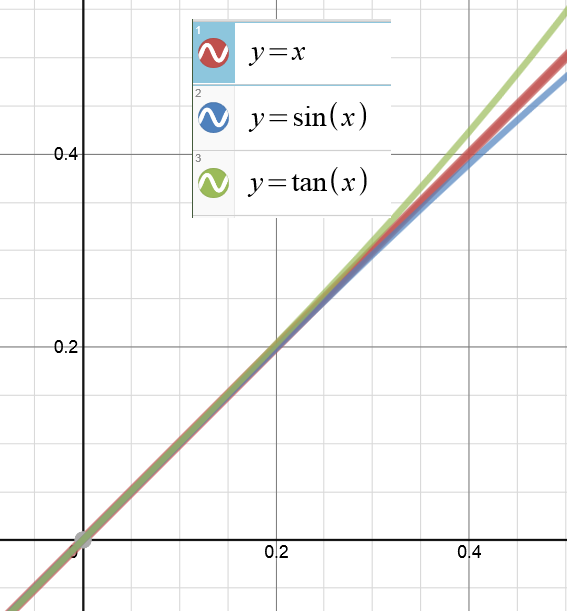Again, if we stay where the angles are small, the three graphs are “equal-ish.”

3. Look at their Taylor Series Representations

This is something that students learn later in their first year of calculus.  These non-polynomial functions can each be expressed as a polynomials with an infinite number of terms.  And they can be approximated with polynomials of finite length.

How these polynomial formulas are developed and how much error you introduce by using only a finite number of terms are topics for several weeks of discussion in calc class.  But for now, let’s look at the first few terms of the Taylor polynomials for the sine function and the tangent function: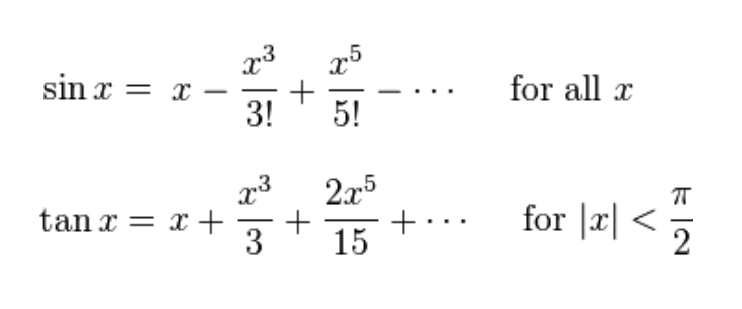Notice that the first term is the same: it’s just ‘x’.  Then, after that, the next term has x-cubed.  But if x is already small, then x-cubed is going to be REALLY small.  Negligibly small.  As we already expect from looking at the charts and graphs, sin(x) is a little bit smaller than x and then tan(x) is a little bigger than x.  But for small values, the differences are negligible.

I know you are probably already convinced. But I have one more reason for you to believe this and it gives me an excuse to re-use one of my favorite diagrams…

4. Look at the Geometry

Here it is again:This was the diagram we used to learn the geometric meaning of all of the trig ratios.  Now I am going to make some changes to the diagram:  I am going to make the angle smaller and also I am going to delete any of the segments we don’t need right now.  Then I am going to zoom in on the part we care about:The length of the blue segment is sin(θ).  The length of the orange segment is tan(θ).  And because it is a unit circle, the length of that intercepted arc is just θ.  (If you are not sure why that is true, think about the definition of radian measure — or read about it in this earlier post here.)

Now look at how the lengths of those two segments compare to the length of the arc.  One of them (the sine) is a little shorter.  One of them (the tangent) is a little longer.  But as the angle gets smaller and smaller, the differences become negligible!

Well, I hope one of these four methods have convinced you that this is legal.  If not, I hope at least that you enjoyed the joke at the beginning.

# Invert and Multiply…but WHY?

Most of my posts so far have addressed high school level math.  This one is for younger students (and their teachers).

At another blog that I follow, Kitchen Table Math, there has been recent discussion about the “invert and multiply” rule for dividing fractions and whether it is important and/or appropriate to teach the reasoning behind this rule.

You know I am going to vote “yes”.  But what kind of derivation should we use here?

The rule can be derived algebraically.  But that doesn’t really help the grade-school students who are just learning it.  It can also be derived in a algebra-ish way.  A commenter at Kitchen Table Math pointed to the explanation found here:

http://www.moveitmaththesource.com/realfractions/mrnovaksproof.html

Mr. Novak’s explanation is elegant and concise. And it certainly answers the question: why do we invert and multiply in order to divide fractions?  But it would be nice if students also had an intuitive feel for why this works.

We can build the rule step-by-step in a manner that helps that intuition to develop.

Step 1: Division is for Sharing

You have 12 cookies – each a pretty good size —  and you need to put them in individual serving bags to hand out at a party.  By deciding how many to put in each bag, you also determine how many serving bags you can make.  For example, you could put 12 cookies in each bag, but then you would only have enough for 1 bag.  On the other hand, it you put 6 cookies in each bag, then you can fill 2 bags because 12/6 = 2. That seems obvious, but it establishes a method: we are going to divide the total number of cookies by the amount in each bag to find how many bags we can fill.  You soon notice that if you put fewer cookies in each bag, you can fill more bags.  For example:

 If we take 12 cookies… And put 12 in each bag… 12 ÷ 12 = 1 We can fill  1 bag If we take 12 cookies… And put 6 in each bag… 12 ÷ 6 = 2 We can fill  2 bags If we take 12 cookies… And put 4 in each bag… 12 ÷ 4 = 3 We can fill  3 bags If we take 12 cookies… And put 2 in each bag… 12 ÷ 2 = 6 We can fill  6 bags If we take 12 cookies… And put 1 in each bag… 12 ÷ 1 = 12 We can fill  12 bags

So yes, dividing by a smaller number gives a bigger answer.  And with 12 cookies, 12 bags is the most you can fill…or is it?  I mentioned that these were pretty big cookies.  Hmmm…

Step 2: Dividing by Fractions (of the “one over” variety)

You decide to put make the serving size a ½-cookie.  Since that serving size is even smaller, you expect to fill more bags.  Though you have not been taught how to divide fractions, you can do this one by thinking:

If I am putting ½ in each bag, then each cookie is enough for 2 bags.  So 12 cookies fill 24 bags.   I guess that must mean 12 ÷ ½ = 24.

Need to fill more bags?  Use smaller portions!  Give everyone 1/3 of a cookie…

You can see where this is going.  Let’s continue the chart…

 If we take 12 cookies… And put 1/2 in each bag… 12 ÷ (1/2) = 24 We can fill  24 bags If we take 12 cookies… And put 1/3 in each bag… 12 ÷ (1/3) = 36 We can fill  36 bags If we take 12 cookies… And put 1/4 in each bag… 12 ÷ (1/4) = 48 We can fill  48 bags If we take 12 cookies… And put 1/5 in each bag… 12 ÷ (1/5) = 60 We can fill  60 bags

The trend has continued.  Dividing by a smaller number gives a bigger answer.  And even if you don’t know the rule for dividing by fractions, you can certainly divide by these fractions!

To divide by “one over anything”, just multiply by the denominator.

STEP 3: Any fraction at all!

Suppose you have those 12 big cookies and you decide to give everyone (2/3) of a cookie.  How many servings can you make?

We can figure this out using the reasoning we followed above, with one extra twist.

We have already seen that if you want to give everyone just (1/3) of a cookie, you will have enough to fill 12 × 3 = 36 bags.  But now you decide to give everyone TWO thirds of a cookie.  But you have already filled all those bags – why not just give everyone two of the bags?  You will have enough for 36 ÷ 2 = 18 servings.

Now go back and look at how we got that answer.  We don’t have to know an official rule to divide the 12 cookies into servings that each have 2/3 of a cookie.  We can just multiply by 3 and then divide by 2, which is the same as multiplying by 3/2.

Let’s try another:

Suppose you have 36 pizza pies to sell and you want to give 3/5 of a pizza to each customer.  (No one cuts pizzas into fifths, but don’t worry about that.)  How many customers can you serve before running out of pizza?

Well, if we give everyone ONE fifth of a pie, we can feed 36 × 5 = 180 customers.  Giving everyone THREE fifths means that we can only serve 180 ÷ 3 = 60 customers.

So to find 36 ÷ (3/5) we multiply by 5 and then divide by 3 which is the same as multiplying by 5/3.

Which derivation is “better”?

If I could only show students one explanation, I would go with Mr. Novak’s.  But if I had time to explore and play, I would start with what you see above.  I like that it encourages number sense and intuition.  Also, it introduces an idea that students will see again many times: that existing patterns can be extended to cover new situations.